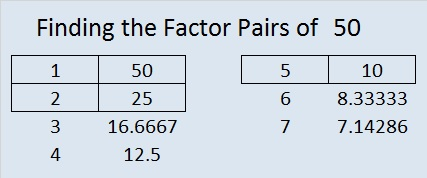# 50 and Multiples

Multiples and factors are related.

For example, 50 is a multiple of all these numbers: 1, 2, 5, 10, 25, and 50.

And 1, 2, 5, 10, 25, and 50 are all factors of 50.

50 is a composite number. 50 = 1 x 50, 2 x 25, or 5 x 10. Factors of 50: 1, 2, 5, 10, 25, 50. Prime factorization: 50 = 2 x 5 x 5, which can also be written 2 x 5².

The first few multiples of 50 are 50, 100, 150, 200, 250, 300, and so on…

If the difference between factors and multiples is confusing, this poster should help. Thanks to Resourceaholic for mentioning this great resource in one of her posts.Sometimes 50 is a clue in the FIND THE FACTORS puzzles. Even though it has other factors, we only use 50 = 5 x 10 to fill in the table.

Each of the clues inside this puzzle are MULTIPLES of a number from 1 to 12. Can you write every number from 1 to 12 in the top row as well as in the first column so that the clues are multiples of the numbers that you write?This week’s puzzles are available in an excel file here. If you have a spreadsheet program on your computer, you can access it. If you enable editing in excel, you can type your answers directly onto the puzzle, and you can also easily print the puzzles.

Here are the factors to last week’s level 4 puzzle:The chart below shows one possible way to arrive logically at the solution.